# 光子灯笼中模式耦合及其对光束质量的影响Mode Coupling in Photonic Lantern and Its Influence on Beam Quality

• 全文下载: PDF(2185KB)    PP.14-21   DOI: 10.12677/OE.2018.81003
• 下载量: 540  浏览量: 1,388

Through simulating the coherence superposition process of the existential mode in the photonic lantern, the beam spot shape, combining efficiency and the changing rule of the beam quality with the phase difference between modes, power ratio and polarization are obtained on the basis of the mode coupling theory and the second moment algorithm of M2 factor. In addition, the factors which need to be considered in controlling the photonic lantern are also analyzed. Research indicates that the output can be of high beam quality and high coupling efficiency only on condition that the input power ratio and phase difference of various mode of photonic lantern are under control at the same time; the needed special spots can be achieved through reasonable control of the input amplitude, phase and polarization.

1. 引言

2. 基本原理

2.1. 光子灯笼结构与模式

2.2. 模式相干叠加的一般理论Figure 1. (a) Photonic lanterns in mode controlling system. (b) Photonic lanterns in astronomical measuring system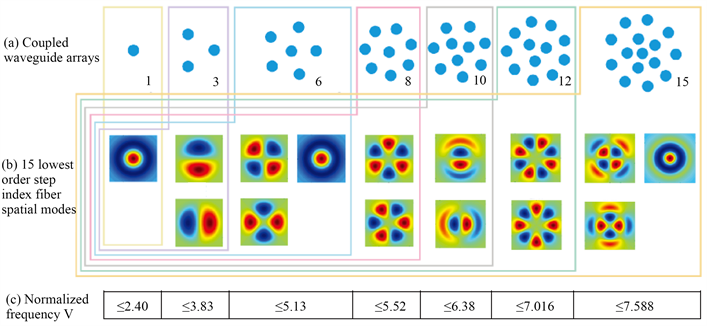Figure 2. (a) Coupled waveguide arrays. (b) 15 lowest order step index fiber spatial modes. (c) Normalized frequency V

$E\left(r,\varphi \right)=\left\{\begin{array}{l}\underset{m,n}{\sum }\frac{\sqrt{{\eta }_{mn}}}{\sqrt{{P}_{mn}}}\frac{{J}_{m}\left({U}_{mn}r/a\right)}{{J}_{m}\left({U}_{mn}\right)}\mathrm{cos}\left(m\varphi \right){\text{e}}^{i{\psi }_{mn}},\text{\hspace{0.17em}}0\le r\le a\\ \underset{m,n}{\sum }\frac{\sqrt{{\eta }_{mn}}}{\sqrt{{P}_{mn}}}\frac{{K}_{m}\left({W}_{mn}r/a\right)}{{K}_{m}\left({W}_{mn}\right)}\mathrm{cos}\left(m\varphi \right){\text{e}}^{i{\psi }_{mn}},\text{\hspace{0.17em}}a\le r\le b\end{array}$ (1)

$\eta =\frac{1}{N}\frac{{|\underset{m=1}{\overset{N}{\sum }}\sqrt{{P}_{m}}{\text{e}}^{j{\theta }_{m}}|}^{2}}{\underset{m=1}{\overset{N}{\sum }}{P}_{m}}$ (2)

2.3. 光束质量M2的理论计算方法

M2因子是一种应用广泛且被大家所接受的光束质量评判参数  ，能够反映光束在传输过程中的质量特性  。20世纪90年代初，A.E.Siegman引入空间频率和强度矩的概念，总结出基于二阶强度矩的M2因子计算方法。以 $x$ 方向为例，设一光束的光场用 $E\left(x\right)$ 表示，则其对应的空间频谱为 $\stackrel{^}{E}\left(s\right)$ ，则该光束的M2因子可表示为：

${M}_{x}^{2}=4\text{π}{\sigma }_{x}{\sigma }_{s}$ (3)

${\sigma }_{x}^{2}=\frac{\underset{-\infty }{\overset{+\infty }{\int }}{\left(x-\stackrel{¯}{x}\right)}^{2}I\left(x\right)\text{d}x}{\underset{-\infty }{\overset{+\infty }{\int }}I\left(x\right)\text{d}x},\text{\hspace{0.17em}}\text{\hspace{0.17em}}{\sigma }_{s}^{2}=\frac{\underset{-\infty }{\overset{+\infty }{\int }}{\left(s-\stackrel{¯}{s}\right)}^{2}\stackrel{^}{I}\left(s\right)\text{d}s}{\underset{-\infty }{\overset{+\infty }{\int }}\stackrel{^}{I}\left(s\right)\text{d}s}$ (4)

$\stackrel{¯}{x}$$\stackrel{¯}{s}$ 分别为空间和频域的重心：

$\stackrel{¯}{x}=\frac{\underset{-\infty }{\overset{+\infty }{\int }}xI\left(x\right)\text{d}x}{\underset{-\infty }{\overset{+\infty }{\int }}I\left(x\right)\text{d}x},\text{\hspace{0.17em}}\text{\hspace{0.17em}}\stackrel{¯}{s}=\frac{\underset{-\infty }{\overset{+\infty }{\int }}s\stackrel{^}{I}\left(s\right)\text{d}s}{\underset{-\infty }{\overset{+\infty }{\int }}\stackrel{^}{I}\left(s\right)\text{d}s}$ (5)

3. 仿真结果及分析

3.1. 耦合结果随影响因素的变化规律

$L{P}_{01}$ 模式和 $L{P}_{11}$ 模式的相干叠加为例，根据式(1)可知， $L{P}_{01}$ 模式和 $L{P}_{11}$ 模式(暂不考虑偏振)相干叠加的光场表达式为：

$E=\left\{\begin{array}{l}\frac{\sqrt{\eta }}{\sqrt{{P}_{01}}}\frac{{J}_{0}\left({U}_{01}r/a\right)}{{J}_{0}\left({U}_{01}\right)}+\frac{\sqrt{1-\eta }}{\sqrt{{P}_{11}}}\frac{{J}_{1}\left({U}_{11}r/a\right)}{{J}_{1}\left({U}_{11}\right)}\mathrm{cos}\left(\varphi \right){\text{e}}^{-i\Delta \phi },\text{\hspace{0.17em}}0\le r\le a\\ \frac{\sqrt{\eta }}{\sqrt{{P}_{01}}}\frac{{K}_{0}\left({W}_{01}r/a\right)}{{K}_{0}\left({W}_{01}\right)}+\frac{\sqrt{1-\eta }}{\sqrt{{P}_{11}}}\frac{{K}_{1}\left({W}_{11}r/a\right)}{{K}_{1}\left({W}_{11}\right)}\mathrm{cos}\left(\varphi \right){\text{e}}^{-i\Delta \phi },\text{\hspace{0.17em}}a\le r\le b\end{array}$ (6)

3.1.1. 模式间相位差对耦合结果的影响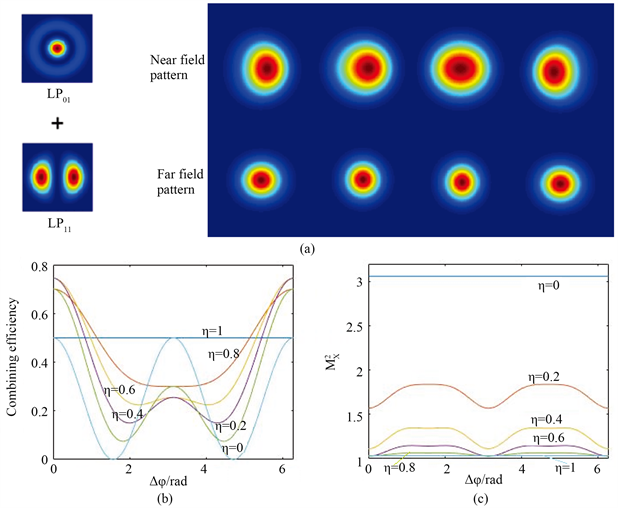Figure 3. (a) Near- or far-field pattern, (b) Δφ-combining efficiency diagram, (c) $\Delta \phi \text{-}{M}_{x}^{2}$ diagram, with the increasing of $\Delta \phi$ when $L{P}_{01}$ and $L{P}_{11}$ coherent

3.1.2. 模式功率占比对耦合结果的影响

3.1.3. 偏振对叠加结果的影响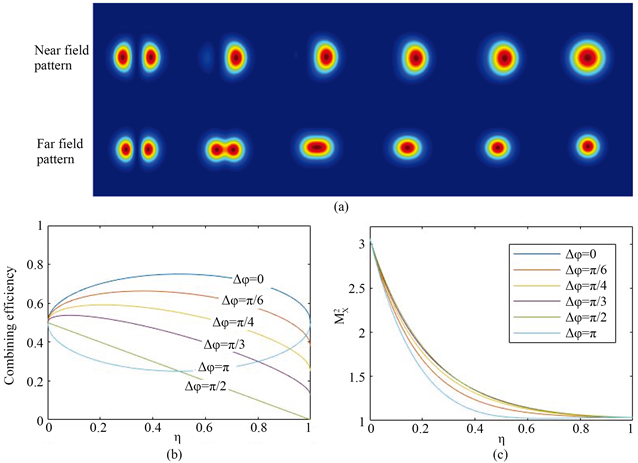Figure 4. (a) Near- or far-field pattern, (b) h-combining efficiency diagram, (c) $\eta \text{-}{M}_{x}^{2}$ diagram, with the increasing of h when $L{P}_{01}$ and $L{P}_{11}$ coherent

3.2. 三通道光子灯笼中的模式控制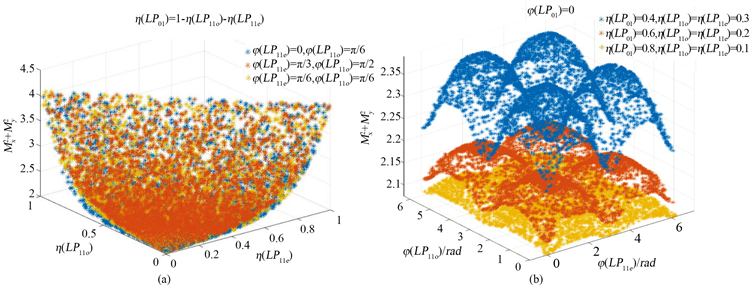Figure 5. (a) $\eta \text{-}{M}^{2}$ diagram, (b) $\Delta \phi \text{-}{M}^{2}$ diagram, when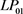, $L{P}_{11e}$ and $L{P}_{11o}$ coherent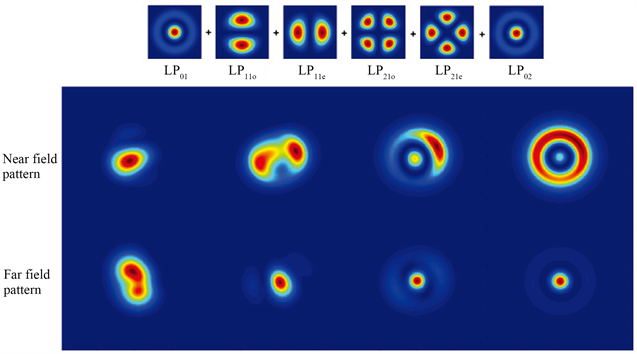Figure 6. Different near- or far-field pattern when the modes coherent in the six-channel photonic lantern

4. 结论

  Leon-Saval, S.G., Argyros, A. and Bland-Hawthorn, J. (2010) Photonic Lanterns: A Study of Light Propagation in Multi-Mode to Single-Mode Converters. Optics Express, 18, 8430-8439. https://doi.org/10.1364/OE.18.008430  Thomson, R.R., Birks, T.A., Leon-Saval, S.G., Kar, A.K. and Bland-Hawthorn, J. (2011) Ultrafast Laser Inscription of an Integrated Photonic Lantern. Optics Express, 19, 5698-5705. https://doi.org/10.1364/OE.19.005698  Montoya, J., Aleshire, C., Hwang, C., Fontaine, N., Velázquez-Benítez, A., Martz, D., Fan, T. and Ripin, D. (2016) Photonic Lantern Adaptive Spatial Mode Control in LMA Fiber Amplifiers. Optics Express, 24, 3405-3413. https://doi.org/10.1364/OE.24.003405  Montoya, J., Hwang, C., Martz, D., Aleshire, C., Fan, T.Y. and Ripin, D.J. (2017) Photonic Lantern kW-Class Fiber Amplifier. Optics Express, 25, 27543-27550  Duocastella, M. and Arnold, C.B. (2012) Bessel and Annular Beams for Materials Processing. Laser & Photonics Reviews, 6, 607-621. https://doi.org/10.1002/lpor.201100031  Fontaine, N.K., Ryf, R., Bland-Hawthorn, J. and Leon-Saval, S.G. (2012) Geome-tric Requirements for Photonic Lanterns in Space Division Multiplexing. Optics Express, 20, 27123-27132. https://doi.org/10.1364/OE.20.027123  Bland-Hawthorn, J. and Kern, P. (2009) Astrophotonics: A New Era for Astronom-ical Instruments. Optics Express, 17, 1880-1884. https://doi.org/10.1364/OE.17.001880  Jovanovic, N., Spaleniak, I., Gross, S., Ireland, M., Lawrence, J.S., Miese, C., Fuerbach, A. and Withford, M.J. (2012) Integrated Photonic Building Blocks for Next-Generation Astronomical Instrumentation I: The Multimode Waveguide. Optics Express, 20, 17029-17043. https://doi.org/10.1364/OE.20.017029  冯国英, 周寿桓, 高春清. 激光模场及光束质量表征[M]. 北京: 国防工业出版社, 2016.  Siegman, A.E. (1997) How to (Maybe) Measure Laser Beam Quality. Optical Society of America Annual Meet-ing.